BASH Programming

# How to use Variables in Bash

For those who have performed some programming tasks before, they will be familiar with variables. But, for those who haven’t any programming knowledge, variables are used to temporarily store a piece of information. Variables are defined in a program to store specific types of data like integer, float, and strings. As we know that bash is a weakly typed language in which variables are not bound with a particular data type, therefore, no need to define any data type to the variable at the declaration time. If we assign a numeric value to a variable then it will take it as an integer and in the case of a text value, it will behave as a string. In Bash Script, variables that can be defined in a Bash file or from the terminal are used to manage and control the actions of the whole bash program. Variables are quite easy to use but, if you don’t have a proper understanding of how they work then, you can easily get yourself in trouble.

In this article, we will discuss a variety of different methods through which we can understand how to create and use variables in a Bash Script. We have executed different examples related to variables on Ubuntu 20.04 Linux distribution. Let’s start the demonstration.

## How do variables work?

As we mentioned above, a variable is a temporary storage for a piece of information.
The following two actions you may perform while using a variable in a bash script:

• Set a particular value for a variable.
• Read value for a variable.

You can value variables using different ways. The most common is to directly set a value to a variable or you may set its value as a result of command processing or a program.
Reading a variable, we need to place its name with the \$ sign at the beginning of that variable that you may want to read. Before executing every line of a Bash script, first, it checks if any variable names are present. It finds each variable and replaces its value with the name of the variable. Then, it starts the execution of a particular line of code and repeats the process for the next line.

There are following some important points on Syntax that you need to follow while reading a variable:

• Don’t use any special character or \$ sign while setting a variable value
• When reading a variable, place a \$ sign at the beginning of the variable name
• Some programmers write variable names in all uppercase but, we can assign names according to our preference. They can be all uppercase, lowercase, or a mixture of both.

You can set and read data from a variable through the terminal in the following way: First, you need to open the terminal application on your system. Open the terminal using the keyboard shortcut by pressing ‘Ctrl + Alt + t’. Or you can open it through your application launcher search bar. Click on ‘Activities’ and then type ‘terminal’ in the search bar that will be displayed on the desktop and press ‘Enter’. You will see the following output on your terminal screen. Click on the terminal icon and launch it.

## The basic syntax of variable declaration; Setting the variable value

As we have discussed earlier in bash, we don’t need to define variable type when you declare a variable. You don’t have to declare a variable. Just assign a value to a variable to reference it.

variable_name=value

For example, we want to assign a value like the string ‘welcome to bash programming: use of variables’ to a variable named ‘var_str’. Using the following command, you can do this task:

var_STR="welcome to bash programming: use of variables"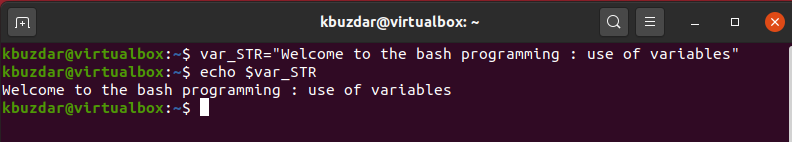Unlike most of the other modern programming languages, bash offers a picky syntax for setting variables. You should be aware that there is no need to add whitespace between the variable name, equal symbol, and the value that you want to assign it. Otherwise, it will throw an error message.

my_var=  “Say hello”

In the above command, you will receive an error due to the whitespace after the equal sign and the assigned value.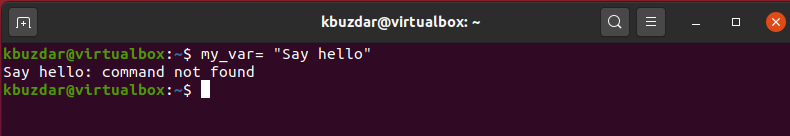## Example: declaration and reading a string using variable

Let’s take an example in which we will store a string “Rules: how to use a variable in bash” and then the variable VALUE is retrieved through the echo command by appending the ‘\$’ sign at the beginning of the variable name. The following command you need to follow to display a string on the terminal:

\$ my_var="Rules: how to use the variable in bash”

\$ echo \$my_var

You will see the following output on the terminal window:If you will not use the ‘\$’ sign then, the program output will show different results and you may not get the required output. Let’s show you the following example:

\$ my_var="Rules: how to use a variable in bash”

\$ echo my_varIn the above command, the ‘\$’ sign is removed with the variable name ‘echo my_var’. So, you will retrieve only the name of the variable on the output.

## Valid variable names

You can assign variable names underscores and sequences of alphanumeric characters.
The variable name should start with alphanumeric characters or an Underscores. It should not be started with a number or digit.

## Examples of variables names

• hello
• n4
• number_my_array
• _num

## Combining two variables output

You don’t need to use any operator to combine two or more variables output like other languages.

## Example

For example, here we are using a \$var1 in which string value to be stored, and \$var2 is used to store any integer or a numeric value. Execute the following commands on the terminal that will combine the var1 and var2 output.

\$ var1="The price of the house is \$"

\$ var2=50

\$ echo \$var1\$var2

The following output will be shown on the terminal after executing the above-mentioned commands:## Important Note:

The output can be printed without using any quotation but, if you want to use quotations then, only you have to use double-quotes.

## Concatenating two variables

Double quotes are used to read the variable’s value in bash.

## Example

Let’s take an example of the concatenation of two variables. We have used double quotes for one echo statement and a single quote for another echo statement. We have executed the following commands on the terminal that you can check the below-mentioned output:

\$ var="variable concatenation in"

\$ echo "\$var Programming"

\$ echo '\$var Programming'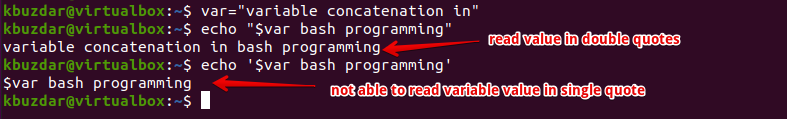In the above screenshot, you can observe that when you have used the double quotes with echo command then, it reads the variable value. In the case of a single quote, it is not able to read the variable value.

## Do arithmetic operations by using variables

In bash programming, we can do different arithmetic tasks like other programming languages. It takes numeric values or integers as strings. However, you can’t perform any arithmetic operation using just normal simple expressions. It only combines the numeric values in that case. Using the double starting and ending brackets with the expression, you can do the arithmetic operations.

## Example

For example, we have a variable n in which 50 numeric values are stored. We want to add more 20 in variable then, using the following command you can perform this operation:

\$ n=50

\$ echo \$n

\$ echo \$n+20

\$ ((n=n+20))

\$ echo \$n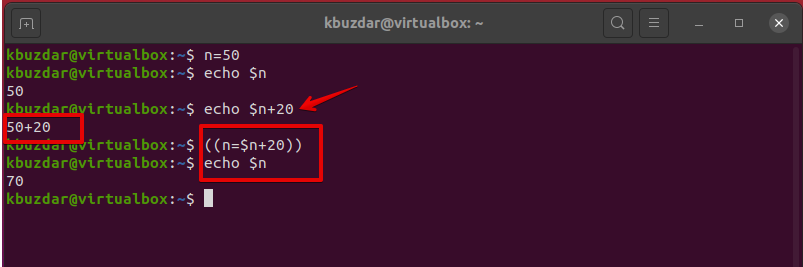In the above command, you have seen \$n+20 command just combine two values. It does not give you the desired output. By adding initial brackets ((n=n+20)), you have performed the arithmetic operation with result 70.

## Do arithmetic operation by using bc command

The other method to do arithmetic operations is the use of bc command in bash.

## Example

Using the following commands on the terminal, you can perform arithmetic tasks:

\$ n=65

\$ echo \$n/10 | bcIn the above code, you can see that when you have used the bc command for doing arithmetic operation division then it omitted the fractional parts from the result.

\$ n=65

\$ echo \$n/10 | bc -lWhen you have used the -l option with bs command, you also get the fractional value as a result.

## Use of variables in a bash script file

You can also initialize a variable in a bash script using the same method which is mentioned in the above examples. However, you need to create a bash script file. To do that, create a file using a text editor and paste the following code in it. Now, save it with .bash or .sh extension.

## Example

In the following script, we have declared two variables one is a string and the other one has numeric values. We want to subtract 10 from the given number. Execute the following script for this purpose:

#!/bin/bash

Str="How to use variables in BASH Script"

# Display string value

echo \$Str

num=100

# Subtract 10 numeric values from a variable num=100

(( result=\$num-10))

# Display the numeric output

echo \$result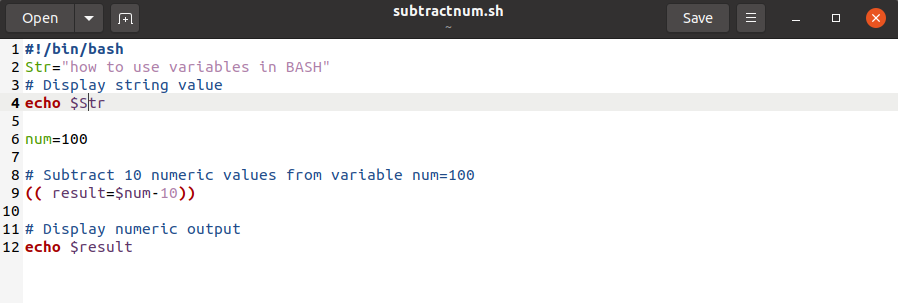You will see the following output on the terminal: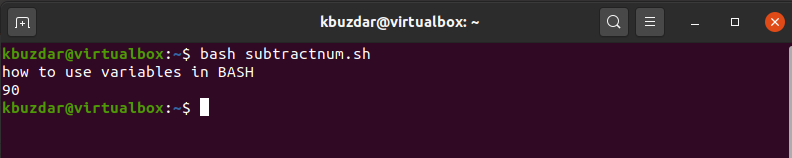## Use of local and global variables

Like other programming languages, you can define local and global variables in bash programming. Let’s elaborate on the concept of local and global variables with an example.

## Example

For example, in the following Bash Script, we have used local and global variables. The following script has one global variable named ‘num’ and two local variables are used named ‘num’ and ‘m’.

We want to add two variable values using a function addition(). When this function will call the value of the local variable ‘num’ is taken for calculation but the number which is a global variable remains unchanged. When we will declare local variables we need to use a local keyword with the variable name.

#!/bin/bash

num=10

{
local num=10
local m=10
(( num=num+m ))
echo \$num
}

echo \$num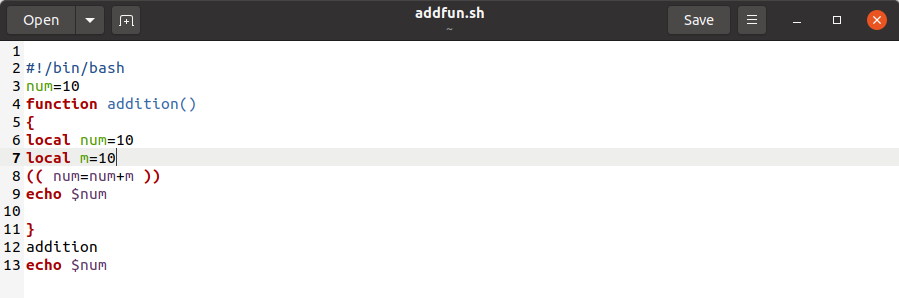Run the above script using the following command:

The following output will be displayed on the terminal after running the above script:## How to use array variables in BASH

Arrays are used to store the list of data. Therefore, in the bash script, we can also use array variables to store data.

## Example

Let’s take an example that will show you how to use array variables in bash script. The arrays elements are separated by spec in Bash programming. Here, we have taken an array of 5 elements. We don’t have any pre-defined function to count the total array’s elements. In bash, # with * or ‘@’ is used to count the total number of array’s elements. All array elements are indicated by * sign. We have used a loop to iterate within the array elements. Then, the script will read the array values and with a key and will print the output on the terminal.

#!/bin/bash

my_arr=(CentOS Ubuntu Debian Linux Mint Solaris MacOS Windows)

# count the total number of elements in an array
total=\${#my_arr[*]}

echo "Total array elements are: \$total"

#display value of each element of an array
echo "Array Elements values:"

for val in \${my_arr[*]}
do
printf "   %s\n" \$val
done

#display each array’s element value with a key
echo "Array Elements values with key:"

for key in \${!my_arr[*]}
do
printf "%4d: %s\n" \$key \${my_arr[\$key]}
done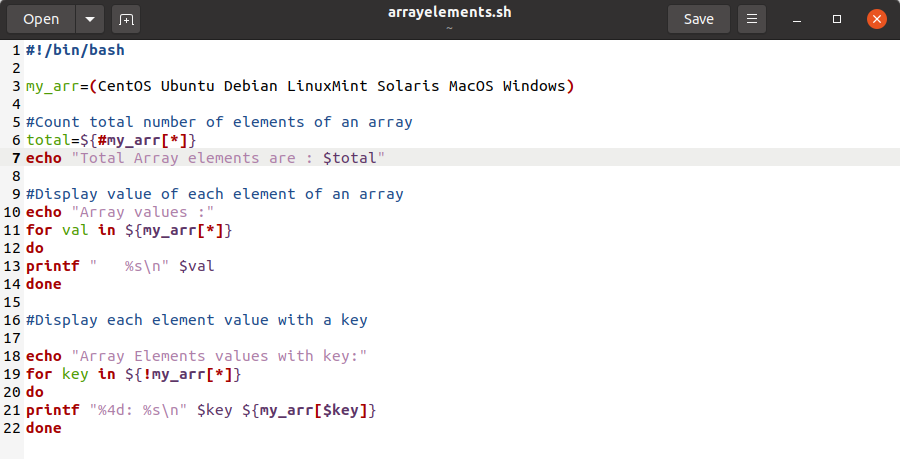The following output will be display on the terminal: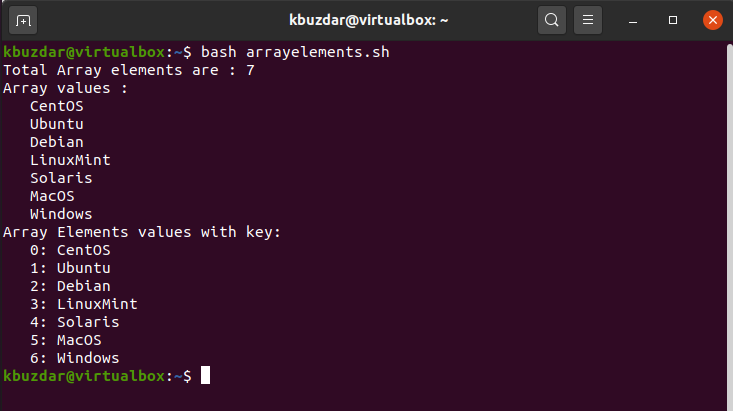We are summarizing some important key point about the variables in Bash programming:

• Variable declaration
variable_name=value

While setting a value for a variable. You must remember that no need to add spaces on either side of the = sign.

• Quotations ” ‘
• Use double quotes for variable substitution, you will not use a single quote for reading a variable.
• Use ((n=n+10)) use initial brackets for arithmetic operations or you can use the bc command.
• Array’s elements you can count using # with * sign.

## Conclusion

In this article, we have explained a clear concept about bash variables that how we can declare and read variables values in bash programming. If you will exercise the above-mentioned examples, you will be able to deal with variables more efficiently in bash scripts. We have executed various commands on the terminal as well as have also executed in a bash script. Bash commands on the command line work exactly the same as in a bash script but when you have a large piece of code you can manage it in a script file rather than running one by one on the terminal.